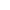# 2023级HAUT新生周赛（四）题解

A.我要成为复数高手

1.当虚数的系数为1/-1时系数省略，

2.当实数部分的系数为0时，实数部分省略。

3.当虚数部分的系数为0时，虚数部分省略。

5.当虚数部分的符号为负数时，加号省略。

```#include<stdio.h>

int main()
{
int t;
scanf("%d",&t);
while(t--)
{
int a, b,c,d,a1,a2;
scanf("%d %d %d %d",&a,&b,&c,&d);
a1 = a + c;
a2 = b + d;
if(a1==0)//实数部分系数为0
{
if(a2==1)
{
printf("i\n");
}
else if(a2==-1)
{
printf("-i\n");
}
else if(a2==0)//虚数系数的特殊情况。
{
printf("0\n");
}
else
{
printf("%di\n",a2);
}
}
else//实数部分为0
{
printf("%d",a1);
if(a2==0)
{
printf("\n");
}
else if(a2==-1)
{
printf("-i\n");
}
else if(a2==1)
{
printf("+i\n");
}//虚数系数的特殊情况。
else
printf("%+di\n",a2);
}
}
return 0;
}

```

B.依稀记得，那是一个烟雨蒙蒙的早晨。

```#include<stdio.h>

int main()
{
int t;
scanf("%d",&t);
printf("%d\n",5*t*t);
return 0;
}

```

C.现在是幻想时刻。

```#include<stdio.h>

int main()
{
int n,k;
scanf("%d %d",&n,&k);
int ans = 0;
int min1 = 1e7;
int x;
for(int i = 0;i<n;++i)
{
scanf("%d",&x);
if(x<=min1)
min1 = x;
if(min1<=k)
if(x-min1>=ans)
ans = x-min1;
}
printf("%d\n",ans);
return 0;
}
```

D 命运之夜

1. 1 1：与运算结果第i位为1，或运算结果第i位为1.
2. 1 0 :与运算结果第i位为0，或运算结果第i位为1.
3. 0 1：与运算结果第i位为0，或运算结果第i位位1.
4. 0 0：与运算结果第i位为0，或运算结果第i为为0.

```#include<stdio.h>

long long arr;
int cnt;//cnt[i]表示有多少数的二进制位中1的个数等于i。
int crr;////crr[i]表示第i个数的二进制位中有多少1。
long long sum;//sum[i]表示有多少数的二进制位中1的个数大于等于i。

int main()
{
int n,k;
scanf("%d %d",&n,&k);
for(int i = 1;i<=n;++i)
{
scanf("%lld",&arr[i]);
int cnt1 = 0;
for(int j = 0;j<=30;++j)//计算每个数中有多少1
{
int c = (1<<j);
if(c&arr[i])
{
cnt1++;
}
}
crr[i] = cnt1;
cnt[cnt1]++;
}
for(int i = 70;i>=0;--i)
{
sum[i] = sum[i+1]+cnt[i];
}
int c = 0;
long long ans = 0;
for(int i = 1;i<=n;++i)
{
int t = k-crr[i];
for(int j = crr[i];j>=0;--j)//将自身减去。保证当前sum[i]表示的是大于i中有多少数的二进制位中1的个数大于等于i。避免重复。
{
sum[j]--;
}
if(t<0)
t = 0;
//cout<<t<<endl;
ans+=sum[t];
}
printf("%lld\n",ans);

return 0;
}
```

E 大富翁！启动！

```#include<stdio.h>
long long arr;
long long dp;
int main()
{
int n;
scanf("%d",&n);
for (int i = 1; i <= n; ++i)
{
scanf("%lld",&arr[i]);
}
for (int i = 1; i <= n+10; ++i)
{
dp[i] = -1e12;
}
for (int i = 1; i <= n + 1; ++i)
{
for (int j = i - 1; j >= 0 && j >= i - 6; --j)
{
if(dp[j]>dp[i])
dp[i] = dp[j];
}
dp[i] += arr[i];
}
printf("%lld\n",dp[n+1]);
return 0;
}
```

F.历经千辛万苦，只为得到你

```#include<stdio.h>
int main()
{
printf("AC!!!\n");
return 0;
}
```

G.火柴的奇怪用途.

```#include<stdio.h>
int main()
{
int a={0},b;
int c={6,2,5,5,4,5,6,3,7,6};//预处理每个个数需要多少火柴。
int ans=0,i,p;
scanf("%d",&b);
for(i=0;i<=2222;i++)
{
p=i;
if(p==0)
a[p] = 6;
while(p>0)//求每个数所用的火柴棒
{
int d = p%10;
a[i]=a[i]+c[d];
p=p/10;
}
}
for(int i=0;i<=1111;i++)
{
for(int j=0;j<=1111;j++)
if(a[i]+a[j]+a[i+j]+4<=b)ans++;//X,Y,Z,加号与等号。
}
printf("%d",ans);
return 0;
}
```

H.奇怪的魔法书

s2串很小，直接暴力匹配即可。

```#include<stdio.h>
#include<string.h>
int main()
{
char arr;
char brr;
scanf("%s %s",arr,brr);
int m = strlen(brr);
int n = strlen(arr);
int ans = 0;
for(int i = 1;i<m;++i)//枚举后缀长度
{
int p = m-i;
char drr ={0};
int cnt = 0;
for(int j = 0;j<i;++j)
{
drr[cnt] = arr[n-i+j];
cnt++;
}
for(int j = 0;j<p;++j)
{
drr[cnt] = arr[j];
cnt++;
}
if(strcmp(drr,brr)==0)
{
ans++;
}
}
printf("%d\n",ans);
return 0;
}
```